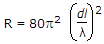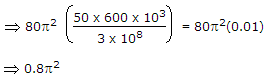# Electronics and Communication Engineering - Electromagnetic Field Theory

16.

Consider a 300 Ω, quarter wave long at 1 GHz transmission line as shown in figure. It is connected to a 10 V, 50 Ω source at one end is left open circuited at the other end. The magnitude of the voltage at the open circuit end of the line is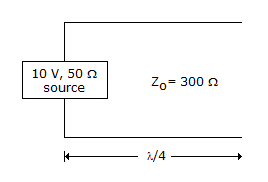A. 10 V B. 5 V C. 60 V D. 60/7 volt

Explanation:

γ = a + jβ a = 0γ = jβ

Propagating wave

v = VI e-rz + VR erz

No change in the voltage at the open end it will same,

vr = 10 volt.

17.

The phase angle corresponding to λ/4 in a standing-wave pattern is

 A. 30° B. 90° C. 135° D. 180°

Explanation: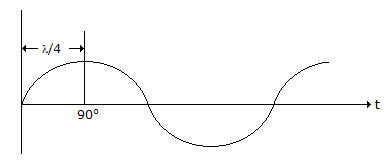18.

A hollow rectangular waveguide has dimensions a = 2b. Calculate the amount of attenuation, if the frequency is 3 GHz, and b = 1 cm.

 A. 49 B. 47.33 C. 50.33 D. 50

Explanation: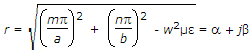Since, wave is attenuated hence β = 0

and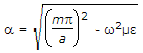Take, dominant mode m = 1,

n = 0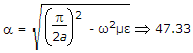.

19.

Calculate the directivity of an antenna for θE = 30°, θH = 60

 A. 46 B. 23 C. 50 D. 100

Explanation: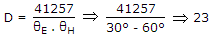.

20.

Most antenna consisting of a 50 meter long vertical conductor operates over a perfectly conducting ground plane. It is base fed at a frequency of 600 kHz. The radiation resistance of the antenna in ohms is

 A.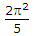B.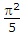C.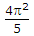D. 20p2

Explanation: